# Abstract Cauchy problem

Jump to: navigation, search

The condensed formulation of a Cauchy problem (as phrased by J. Hadamard) in an infinite-dimensional topological vector space. While it seems to have arisen between the two World Wars (F. Browder in [a2], Foreword), it was apparently introduced as such by E. Hille in 1952, [a2], Sec. 1.7.

Narrowly, but loosely speaking, the abstract Cauchy problem consists in solving a linear abstract differential equation (cf. also Differential equation, abstract) or abstract evolution equation subject to an initial condition. More precise explanations slightly differ from textbook to textbook [a2], [a5]. Following A. Pazy [a5], given a linear operatoron a Banach spacewith domainand given an element, one tries to solve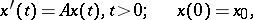i.e., one looks for a continuous functionon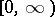such thatis differentiable on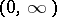,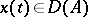for all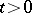, andfor all.

Sinceis required to be continuous at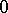, the Cauchy problem can only be solved for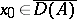.

A Cauchy problem is called correctly set if the solutionis uniquely determined by the initial datum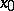. It is called well-posed (properly posed) if, in addition, the solutiondepends continuously on the initial datum, i.e., for everythere exists some constant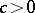(independent of) such that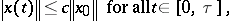and allfor which a solution exists. Sometimes it is also required that solutions exist for a subspace of initial data which is large enough in an appropriate sense, e.g., dense in.

The notion of a Cauchy problem can be extended to non-autonomous evolution equations [a2], [a5] and to semi-linear [a5], quasi-linear [a5], or fully non-linear evolution equations [a1], [a4]. In this process it may become necessary to replace classical solutions by more general solution concepts (mild solutions [a1], limit solutions [a4], integral solutions (in the sense of Ph. Bénilan; [a4]) in order to keep the problem meaningful. See [a1] and the references therein.

Well-posedness of linear Cauchy problems is intimately linked to the existence of-semi-groups of linear operators (cf. also Semi-group of operators), strongly continuous evolution families [a2], [a5] and related more general concepts like distribution semi-groups, integrated semi-groups, convoluted semi-groups, and regularized semi-groups, while the well-posedness of non-linear Cauchy problems is linked to the existence of non-linear semi-groups (the Crandall–Liggett theorem and its extensions) or (semi-) dynamical systems [a1], [a4], and to (evolutionary) processes and skew product flows [a3].